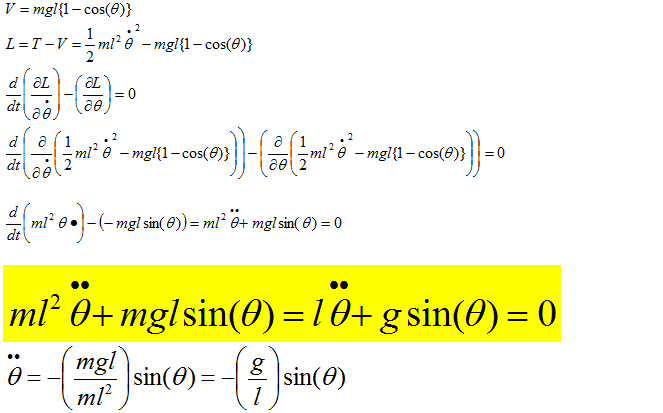### A simple pendulum consisting of a mass suspended in the vertical plane by a massless string.

A simple pendulum is consist of a 0.25 kg mass suspended in the vertical plane by a massless string of 0.5 m long. Assume that the pendulum moves in a plane. We need to numerically simulate the motion of the pendulum if it is released from rest with the string at 3 degrees from the vertical. We also need to plot the angle that the string makes with the vertical as a function of time. On the same axes, we need to plot the analytically solution for the angle obtained by linearizing the equations of motion assuming small oscillations.

### Deriving Equations of motion:### Linearizing the equation of motion by assuming small motion:

We have used three methods to plot the responses. Using Visual Basic's code in excel, using MatLAB code, and MatLAB using ode45 (ordinary differential equation)

### Numerical Solution vs. Theoretical Solution by Excel using Visual Basic in time steps of 0.00001 sec and step report of 0.1 sec

<!--[if !vml]--><!--[endif]-->

 tstep 0.00001 t θ θ dot θ calc θ dot calc g 9.81 0 0.052359878 0 0.052359878 0 l 0.5 0.1 0.047309471 -0.099363422 0.047306808 -0.099403607 0.2 0.033130848 -0.179569434 0.033122908 -0.179621022 step 0.1 0.3 0.012560726 -0.225132799 0.012545855 -0.225169238 0.4 -0.010435845 -0.227254355 -0.010452712 -0.227256856 0.5 -0.031416854 -0.185522546 -0.031433769 -0.185480938 0.6 -0.046336661 -0.10800505 -0.046347699 -0.107904781 0.7 -0.052317983 -0.00966367 -0.052315919 -0.009501595 0.8 -0.048206739 0.090551683 -0.048186484 0.090735523 0.9 -0.03479602 0.173302551 -0.03475643 0.173459503 1 -0.014671658 0.222630468 -0.014617932 0.222703537 1.1 0.008286473 0.229000974 0.008342018 0.228962885 1.2 0.029643814 0.191179991 0.029691849 0.191029412 1.3 0.045283128 0.116471554 0.045310767 0.116224772 1.4 0.052185174 0.019311907 0.052184115 0.018987236 1.5 0.049020361 -0.081581489 0.04898525 -0.081915084 1.6 0.036397484 -0.166747352 0.036331592 -0.167006725 1.7 0.016752931 -0.219746566 0.016665464 -0.219863891 1.8 -0.006126974 -0.230346508 -0.006217316 -0.23028446 1.9 -0.027822822 -0.196494403 -0.027900073 -0.196257126 2 -0.044152002 -0.124728309 -0.044197753 -0.124349608

#### Numerical simlation vs. theoretical solution using MATLAB

clear all

% l*(theta doubledot)+g*theta=0 .... (thtea doubledot)=-g/l*theta
%[z1dot;z2dot] =[z2; -g/l*theta]

clear all
m=.25;
g=9.81;
l=0.5;
defn=inline('[z2;-9.81*0.25*.5/(.5^2*.25)*sin(z1)]','t','z1','z2');
a=0; b=2; N=2/0.001;
h=(b-a)/N; t=a+h*(0:N); LT=length(t);
x0=3*pi/180; y0=0;
w(:,1)=[x0;y0];
w(:,2)=[x0;y0];
for j=2:LT
w(:,j)=w(:,j-1)+h*defn(t(j-1),w(1,j-1),w(2,j-1));
end
z1=w(1,:);
z2=w(2,:);
thetat=3*pi/180*cos(sqrt(9.81/.5)*t);
xt=thetat
figure(1)
plot(t,z1,'b',t,xt,'r--')
xlabel('t -sec')
legend('\theta','\theta true')
title('Numerical Simulation vs. theoretical calculation')
grid on
figure(2)
plot(t,z2,'g',t,yt,'r--')
xlabel('t -sec')
legend('\theta_d_o_t','\theta_d_o_t true')
title('Numerical simulation of velocity vs. theoretical solution')
grid on



#### Numerical solution using MATLAB ode45



function zprime = ME716_6_1(t,z);
%SECONDODE: Computes the derivatives of y 1 and y 2,
%as a colum vector

g=9.81; l=0.5;

zprime = [z(2); -g/l*sin(z(1))];

The top and bottom part of this code from this section should be saved into different m-files

T=[0:0.1:2];
Z0=[85*pi/180 0]';

[t,z]=ode45(@ME716_6_1,T,Z0)

g=9.81
l=0.5
m=0.25

theta=85*pi/180*cos(sqrt(g/l)*t)
tdot=-85*pi/180*sqrt(g/l)*sin(sqrt(g/l)*t)
figure(1)
plot(t,z(:,1),'r',t,theta,'b--')
title('Numerical simulation vs. theoretical calc.')
xlabel('time t sec')
legend('\theta Numerical', '\theta calc.')
grid on

figure(2)
plot(t,z(:,2),'r',t,tdot,'b--')
title('Numerical simulation vs. theoretical calc.')
xlabel('time t sec')
legend('\theta_d_o_t Numerical', '\theta_d_o_t calc.')
grid onNote: When you want to change IVP (initial value problem) in the angle, just change the initial angle where you want to release the pendulum from the rest.

The rights to the contents of this post is reserved to Solid Mechanics.